# HSSlive: Plus One & Plus Two Notes & Solutions for Kerala State Board

## इयत्ता नववी विज्ञान धडा प्रकाशाचे प्रतिबिंब मराठी स्वाध्याय PDF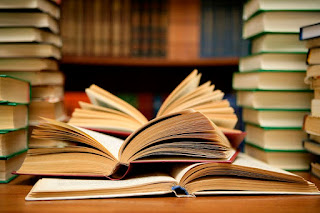इयत्ता नववी विज्ञान धडा प्रकाशाचे प्रतिबिंब मराठी स्वाध्याय PDF

या लेखात, आम्ही विज्ञान धडा प्रकाशाचे प्रतिबिंब विषयासाठी इयत्ता नववी मराठी सोल्यूशन्स देऊ. इयत्ता नववी मधील विद्यार्थी पाठ्यपुस्तकांमध्ये उपस्थित असलेल्या व्यायामांसाठी प्रश्न आणि उत्तरे डाउनलोड आणि कॉपी करण्यास सक्षम असतील.

इयत्ता नववी विज्ञान धडा प्रकाशाचे प्रतिबिंबाच्या पुस्तकात महाराष्ट्र बोर्डाच्या अभ्यासक्रमातील सर्व प्रश्नांचा समावेश आहे. येथे सर्व प्रश्न पूर्ण स्पष्टीकरणासह सोडवले आहेत आणि डाउनलोड करण्यासाठी विनामूल्य उपलब्ध आहेत. महाराष्ट्र बोर्ड इयत्ता नववी विज्ञान धडा प्रकाशाचे प्रतिबिंबाचे पुस्तक खाली दिले आहे. आम्‍हाला आशा आहे की आमच्‍या इयत्‍ता नववी वीच्‍या विज्ञान धडा प्रकाशाचे प्रतिबिंबाचे पुस्‍तक तुमच्‍या अभ्यासात मदत करेल! जर तुम्हाला आमचे इयत्ता नववी चे पुस्तक आवडले असेल तर कृपया ही पोस्ट शेअर करा.

## इयत्ता नववी विज्ञान धडा प्रकाशाचे प्रतिबिंब स्वाध्याय

 मंडळाचे नाव Maharashtra Board ग्रेडचे नाव नववी विषय विज्ञान धडा प्रकाशाचे प्रतिबिंब वर्ष 2022 स्वरूप PDF/DOC प्रदाता hsslive.co.in अधिकृत संकेतस्थळ mahahsscboard.in

## समाधानासह महाराष्ट्र बोर्ड आठवा स्वाध्याय कसे डाउनलोड करायचे?

महाराष्ट्र बोर्ड नववी स्वाध्याय PDF डाउनलोड करण्यासाठी खालील स्टेप्स फॉलो करा:

1. वेबसाइट- Hsslive ला भेट द्या. 'स्वाध्याय' लिंकवर क्लिक करा.
2. महा बोर्ड नववी स्वाध्याय PDF पहा.
3. आता महाराष्ट्र बोर्ड नववी स्वाध्याय तपासा.
4. डाउनलोड करा आणि भविष्यातील संदर्भांसाठी जतन करा.

## इयत्ता नववी विज्ञान धडा प्रकाशाचे प्रतिबिंब स्वाध्याय उपाय

इयत्ता नववी स्वाध्याय मधील विद्यार्थी खालील लिंक्सवरून विज्ञान धडा प्रकाशाचे प्रतिबिंबाचे उपाय डाउनलोड करू शकतील.

a. Explain the difference between a plane mirror, a concave mirror and a convex mirror with respect to the type and size of the images produced.

 Plane mirror Concave mirror Convex mirror Type of image Virtual and Erect Virtual (erect) as well as Real (inverted) Virtual and Erect Size of image Same size Diminished, Same size and magnified Diminished

b. Describe the positions of the source of light with respect to a concave mirror in
1. Torch light
2. Projector lamp
3. Floodlight
(a) Torch light: The source of light is placed at the focus.
(b) Projector lamp : The source of light is placed at the centre of curvature.
(c) Flood light : The source of light is placed just beyond the centre of curvature.

c. Why are concave mirrors used in solar devices?

• Solar devices like solar cooker or solar water heater use solar energy to cook food or heat water.
• When sun rays fall on the concave mirror, they converge and come together in the focal plane.
• Due to convergence, the intensity of sun rays increases and the food or water is heated faster. Hence, concave mirrors are used in solar- devices.

d. Why are the mirrors fitted on the outside of cars convex?

• A convex mirror is used as rear view mirror because they form erect, virtual, and diminished images.
• This, allows the driver to view a large area in a small mirror.

e. Why does obtaining the image of the sun on a paper with the help of a concave mirror burn the paper?

• When sunrays fall on the concave mirror, they converge and come together in the focal plane.
• Due to convergence, the intensity of sunrays increases.
• Hence, image of the sun on a paper with the help of concave mirror bums the paper.

f. If a spherical mirror breaks, what type of mirrors are the individual pieces?

• When a spherical mirror breaks into smaller pieces, the radius of curvature and focal length does not change.
• Hence, it will continue to behave like a spherical mirror only.

2. What sign conventions are used for reflection from a spherical mirror?
According to the Cartesian sign convention, the pole of the mirror is taken as the origin. The principal axis is taken as the X-axis of the frame of reference. The sign conventions are as follows.

1. The object is always kept on the left of the mirror. All distances parallel to the principal axis are measured from the pole of the mirror.
2. All distances measured towards the right of the pole are taken to be positive, while those measured towards the left are taken to be negative.
3. The distance measured vertically upwards from the principal axis are taken to be positive.
4. The distance measured vertically downwards from the principal axis are taken to be negative.
5. The focal length of a concave mirror is negative while that of a convex mirror is positive.

3. Draw ray diagrams for the cases of images obtained in concave mirrors as described in the table on page 122.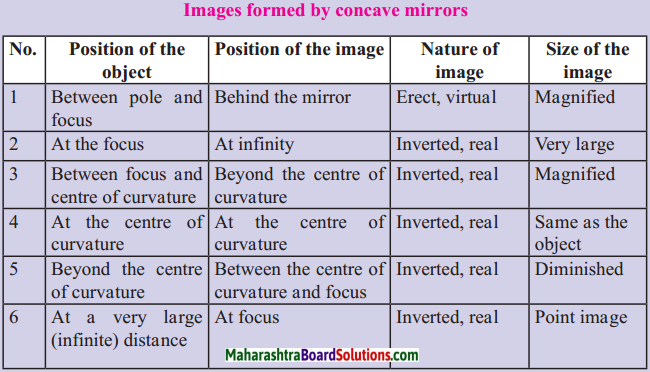(a) A ray diagram for object at infinity for a concave mirror.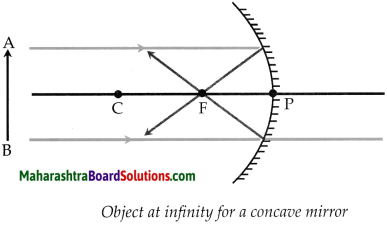Image position Nature of image At focus Real, inverted and point image

(b) A ray diagram for object beyond centre of curvature for a concave mirror.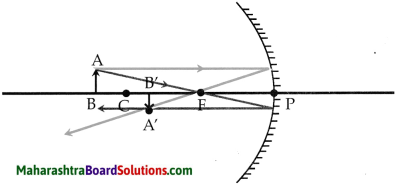An object beyond centre of curvature for a concave mirror

 Image position Nature of image Between the centre of curvature and focus. Real, inverted and diminished.

(c) A ray diagram for object at the centre of curvature for a concave mirror.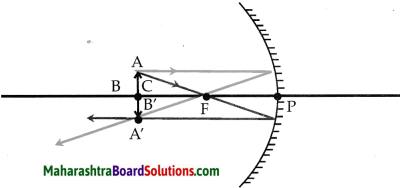Object at centre of Curva fu re be a concave mirror.

 Image position Nature of image At the centre of curvature. Real, inverted and same size

(d) A ray diagram for object between F and C for a concave mirror.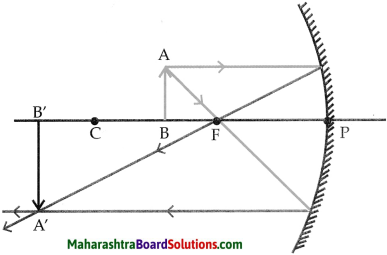Object between F & C for a concave mirror

 Image position Nature of image Beyond the centre of curvature. Real, inverted and magnified.

(e) A ray diagram for obj ect at focus for a concave mirror.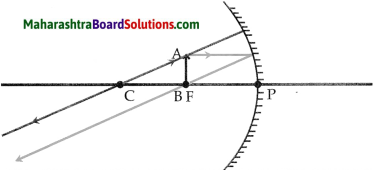Object at focus for a concave mirror.

 Image position Nature of image At infinity. Real, inverted and highly magnified.

(f) A ray diagram for object between pole and focus for a concave mirror.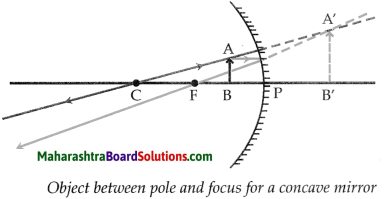Image position Nature of image Behind the mirror. Virtual, erect and magnified.

4. Which type of mirrors are used in the following?
Periscope, floodlights, shaving mirror, kaleidoscope, street lights, headlamps of a car.

 Objects Type of Mirror Periscope Plane mirror Floodlights Concave mirror Shaving mirror Concave mirror Kaleidoscope Plane mirror Street lights Convex mirror Head lamps of car Concave mirror

5. Solve the following examples

a. An object of height 7 cm is kept at a distance of 25 cm in front of a concave mirror. The focal length of the mirror is 15 cm. At what distance from the mirror should a screen be kept so as to get a clear image? What will be the size and nature of the image?
Solution:
Given: Object size (h1) = 7 cm
Object distance (u) = -25 cm
Focal length (f) = -15cm
To find: Image distance (u) = ?
Image size (h2) = ?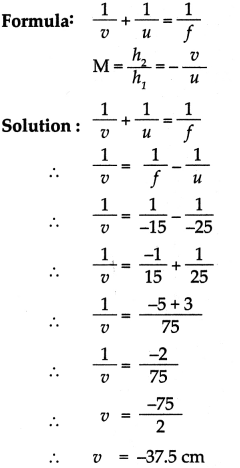The screen should be kept 373 cm in front of the mirror. The image is real.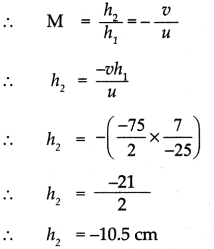The height of the image is 10.5 cm, it is an inverted and enlarged image.

b. A convex mirror has a focal length of 18 cm. The image of an object kept in front of the mirror is half the height of the object. What is the distance of the object from the mirror?
Solution:
Given: Image size (h2) = 1/h1
Focal length (f) = 18 cm
To find: Object distance (u) ?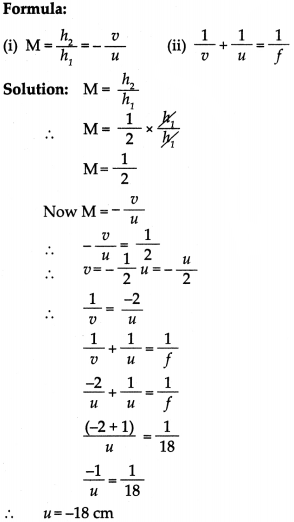The object is placed in front of the convex mirror at a distance of 18 cm.

c. A 10 cm long stick is kept in front of a concave mirror having focal length of 10 cm in such a way that the end of the stick closest to the pole is at a distance of 20 cm. What will be the length of the image?
Solution:
Given: Object size (h1) = 10 cm
Object distance (u) = -20 cm
Focal length (f) = -10 cm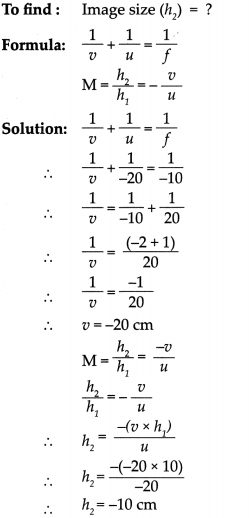The height of the image is 10 cm and it is a real and inverted image.

6. Three mirrors are created from a single sphere. Which of the following:
pole, centre of curvature, radius of curvature, principal axis – will be common to them and which will not be common?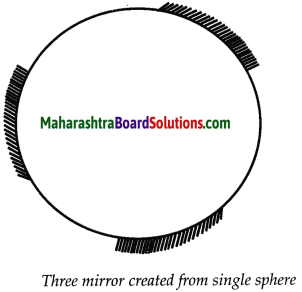• Centre of curvature and Radius of curvature will be common for all three pieces.
• Pole and Principal axis will not be common.

Class 9 Science Chapter 11 Reflection of Light Intext Questions and Answers

Class 9 Science Chapter 11 Reflection Of Light Question 1.
What is light
Light is a form of electromagnetic radiation that produces the sensation of vision.

9th Class Science Chapter 11 Reflection Of Light Exercise Question 2.
What is a mirror?
A mirror is a reflecting surface which reflects light and creates clear images.

9th Class Science Chapter 11 Reflection Of Light Exercise Answer Question 3.
Principal Focus of Concave and Convex Mirror.

 Principal Focus of the Concave Mirror Principal Focus of the Convex Mirror (i) Incident rays which are parallel to the principal axis of a concave mirror, after reflection from the mirror, meet at a particular point in front of the mirror on the principal axis. This point (F) is called the principal focus of the concave mirror.(ii) It is formed in front of the mirror.(iii) Focus of concave mirror is real. (i) Incident rays parallel to the principal axis, after reflection, appear to come from a particular point behind the mirror lying along the principal axis. This point is called the principal focus of the convex mirror.(ii) It is formed behind the mirror.(iii) Focus of convex mirror is virtual.

9th Class Science Chapter 11 Reflection Of Light Notes Question 4.
If we hold a page of a book in front of a mirror, we see laterally inverted letters in the mirror. Why does it happen?

• When we hold a page of a book in front of the mirror, the image of the words appear laterally inverted.
• The image of every point of the word is formed behind the mirror at the same distance from the mirror
• Because of this the left and right side of the image is interchanged.
• Hence, if we hold a page of a book in front of a mirror, we see laterally inverted letters in the mirror.

11 Reflection Of Light Exercise Question 5.
Which letters of the English alphabet form images that look the same as the original letters?
A, H, I, M, O, T, U, V, W, X, Y

9th Science Chapter 11 Reflection Of Light Exercise Question 6.
When a person stands in front of a plane mirror, how is the image formed? What is the nature of the image?

• The image of a person is formed from every point of the source, thereby forming an extended image of the whole source.
• The image formed would be virtual, upright and left-right reversed.

Reflection Of Light Class 9 Questions And Answers Question 1.
Place two plane mirrors at an angle of 90a to each other. Place a small object between them. Images will be formed in both mirrors. How many images do you see? Now change the angle between the mirrors as given in the following table and count the number of images each time. How is this number related to the measure of the angle?
The Relation between the angle between the mirrors and the number of images formed is given by
𝑛=360∘A−1
n = number of images,
A = angle between the mirrors

 Angle Number of images 120° 2 90s 3 60® 5 45® 7 30® 11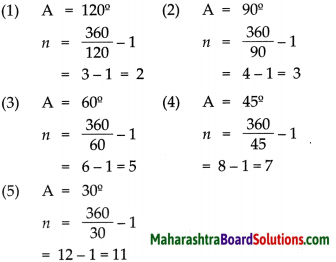Class 9 Science Chapter 11 Reflection of Light Additional Important Questions and Answers

Can you recall?

9th Class Science Chapter 11 Reflection Of Light Question 1.
What is meant by reflection of light and what are the types of reflection?
The bouncing back of light when it hits an opaque surface is called reflection of light. The two types of reflection are regular and irregular reflection.

Reflection Of Light Class 9 Exercise Answers Question 2.
What are the laws of reflection.

• The incident ray, reflected ray and normal all lie in the same plane at the point of incidence.
• The angle of incidence is equal to the angle of reflection.
• The incident ray and the reflected ray lie on opposite sides of the normal.

Choose and the correct option:

Class 9th Science Chapter 11 Reflection Of Light Question Answer Question 1.
If the reflected rays do not actually meet, such an image is called as image.
(a) real
(b) virtual
(c) magnified
(d) inverted
(b) virtual

Class 9 Science Chapter 11 Reflection Of Light Exercise Solutions Question 2.
In a plane mirror, the perpendicular distance of the image from the mirror is equal to
(a) the perpendicular distance of the source from the object.
(b) the perpendicular distance of the source from the mirror.
(c) the parallel distance of the source from the object.
(d) the parallel distance of the source from the mirror.
(b) the perpendicular distance of the source from the mirror

Reflection Of Light Class 9 Notes Pdf Maharashtra Board Question 3.
The image formed in a convex mirror is always
(a) virtual, smaller and behind the mirror
(b) virtual, smaller and in front of the mirror
(c) real, smaller and behind the mirror
(d) real, smaller and in front of the mirror
(a) virtual, smaller and behind the mirror

Reflection Of Light Class 9 Solutions Question 4.
images can be displayed on a screen.
(a) Virtual
(b) Real
(c) Virtual and erect
(d) Virtual and inverted
(b) Real

9th Class Science Chapter 11 Reflection Of Light Exercise Pdf Question 5.
A concave mirror is also called as a mirror.
(a) converging
(b) diverging
(c) plane
(d) outward curved
(a) converging

9th Science Chapter 11 Reflection Of Light Question 6.
The centre of the mirror surface is called its
(a) pole
(b) centre of curvature
(c) principal axis
(d) focus
(a) pole

Class 9 Science Chapter 11 Question Answer Reflection Of Light Question 7.
According to the new sign convention, the of the mirror is taken as origin.
(a) focus
(b) pole
(c) optical centre
(d) centre of curvature
(b) pole

Class 9 Science Chapter 11 Reflection Of Light Exercise Question 8.
A convex mirror is also called as a mirror.
(a) converging
(b) plane
(c) diverging
(d) inward curved
(c) diverging

Reflection Of Light Class 9 Maharashtra Board Question 9.
In order to see the full image of a person standing in front of a mirror, the minimum height of the mirror must be
(a) same height as that of the person
(b) double the height of the person
(c) half the height of the person
(d) quarter the height of the person
(c) half the height of the person

Reflection Of Light Exercise 9th Class Question 10.
If the inner surface of the spherical mirror is reflecting, then it is a mirror, and if the outer surface is reflecting then it is mirror.
(a) convex, concave
(b) convex, plane
(c) concave, plane
(d) concave, convex
(d) concave, convex

9th Std Science Chapter 11 Reflection Of Light Question 11.
The image formed by a concave mirror is
(a) always virtual and erect
(b) always virtual and inverted
(c) virtual if the object is placed between the pole and the focus
(d) virtual if the object is beyond the focus
(c) virtual if the object is placed between the pole and the focus

Question 12.
No matter how far you stand from a spherical mirror, your image appears erect. The mirror may be
(a) plane
(b) concave
(c) convex
(d) either plane or convex
(d) either plane or convex

Question 13.
In case of a concave mirror, an erect image is
(a) real and enlarged
(b) real and diminished
(c) virtual and diminished
(d) virtual and enlarged
(d) virtual and enlarged

Question 14.
A rear view mirror of a car is
(a) plane mirror
(b) concave mirror
(c) convex mirror
(d) cylindrical mirror
(c) convex mirror

Question 15.
An image of an object placed at infinite distance from a concave mirror is formed at
(a) the focus of the mirror
(b) behind the mirror
(c) centre of curvature
(d) infinity
(a) the focus of the mirror

Question 16.
A ray of light parallel to principal axis after reflection from concave mirror passes through
(a) centre of curvature
(b) focus
(c) pole
(d) optical centre
(b) focus

Question 17.
The image made by a plane mirror is a image.
(a) real
(b) virtual
(c) inverted
(d) diminished
(b) virtual

Question 18.
The size of the image of an object placed at the focus of a concave mirror is
(a) erect
(b) very large
(c) same size
(d) diminished
(b) very large

Question 19.
For virtual images, the height is while for real images, it is
(a) positive, positive
(b) negative, positive
(c) negative, negative
(d) positive, negative
(d) positive, negative

Find the odd man out:

Question 1.
Torches, flood lights, head lamps of vehicles, rear view mirror.
Rear view mirror – In rear view mirrors, convex . mirror is used. Concave mirrors are used in the rest.

Question 2.
Side mirrors of cars, parking mirrors, flood lights, mirror fitted in shops.
Flood lights – In flood lights concave mirror is used. Convex mirrors are used in the rest.

Question 3.
Virtual and enlarged, virtual and diminished, real and inverted, real and magnified
Virtual and diminished type of image is not formed by a concave mirror. All the other types of images are formed by a concave mirror.

Question 4.
Image is laterally inverted, image is of same size, image is at same distance, image is diminished.
Image is diminished is not a characteristic of image formed in a plane mirror. Rest of them are characteristics of plane mirror.

Answer the following in one sentence:

Question 1.
What kind of mirror will a doctor use to concentrate on teeth, eyes, ears etc.?
The doctor will use a concave mirror to concentrate on teeth, eyes, ears etc.

Question 2.
What do the nature, position and size of the image depend on?
The nature, position and size of the image depend upon the distance of the object from the reflecting surface.

Question 3.
Give the expression for mirror formula.
1𝑣+1𝑢=1𝑓

Question 4.
State any four uses of concave mirror.
Concave mirrors are used in torches, headlights, shaving mirrors, dentists’ mirrors, solar devices etc.

Question 5.
What are the two types of spherical mirror?
Convex mirror and concave mirror are the two types of spherical mirror.

Match the columns:

Question 1.

 Column ‘A’ Column ‘B’ (1) Plane mirror (a) Rear view mirror (2) Concave mirror (b) At laughing gallery (3) Convex mirror (c) At a hair dresser (4) Irregular   curved mirror (d) At a dentist

(1 – c),
(2 – d),
(3 – a),
(4 – b)

Question 2.

 Column ‘A’ Column ‘B’ (1) Plane mirror (a) Virtual and diminished image (2) Concave mirror (b) Virtual and same size image (3) Convex mirror (c) Real and inverted image

(1 – b),
(2 – c),
(3 – a)

State whether the following statements are true or false. Correct the false statements:

(1) If the mirrors are kept at right angle to each other, then the number of images formed will be 4.
(2) A convex mirror is used in flood lights.
(3) A concave mirror always forms a magnified image.
(4) Images formed by convex mirrors are always virtual.
(5) The distance between the focus and the pole is called the radius of curvature.
(6) Reflection from a spherical mirror obeys laws of reflection.
(7) The reflecting surface of a concave mirror is curved.
(8) Distances measured in the direction of the incident light are taken as positive.
(9) If the image is erect, the height of the image is negative.
(10) A real image can be displayed on a screen.
(11) A concave mirror always forms a real and inverted image.
(12) Doctors use diverging beam of light to study teeth, ears and eyes.
(1) False. if the mirrors are kept at right angle to each other then the number of images formed will be 3.
(2) False. a concave mirror is used in flood lights.
(3) False. a concave mirror can sometimes form a diminished image as well.
(4) True
(5) False. the distance between the focus and the pole is called the focal length.
(6) True
(7) True
(8) True
(9) False. if the image is erect, the height of the image is positive.
(10) True
(11) False. a concave mirror can also form a virtual and erect image.
(12) False. doctors use a converging beam of light to study teeth, ears and eyes.

Give scientific reasons:

Question 1.
A concave mirror is called a converging mirror.

• When rays of light parallel to the principal axis are incident on concave mirror, they converge.
• After convergence, they meet at one point on the principal axis, hence concave mirror is called converging mirror.

Question 2.
Concave mirrors are used in torches and in car headlights.

1. Concave mirrors are used in torches and car headlights because when a source of light is placed at the focus of a concave mirror, a parallel beam of light rays is obtained.
2. This helps us to see things upto a considerable distance in the darkness.

Question 3.
A dentist uses a concave mirror while examining teeth.

• A concave mirror produces an erect, virtual and magnified image of an object placed between its pole and focus.
• A dentist uses this principle to get a clear and distinct image of teeth, hence, a dentist uses a concave mirror.

Solve the following numerlcals.

Tips for solving numerical:

• Object distance (u) is always -ve
• If Image distance (u) is +ve then image is behind the mirror and virtual. if u is -ve then image is in front of the mirror and real.
• Object height (h1) is always +ve since it is erect.
• Image height (h2) can be +ve for virtual and -ve for real.

Type – A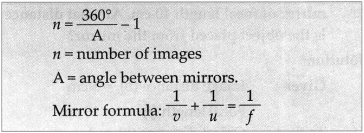Question 1.
A bird is sitting in front of two plane mirrors inclined at an angle of 600 to each other. How many images does the bird see in the mirror?
Solution:
Given : Angle between mirror A = 600
To find: Number of images formed n = ?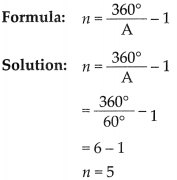The brrd sees 5 images in the mirror.

Question 2.
A coin is kept in front of two plane mirrors inclined to each other. If 3 images of the coin are seen then what is the angle A between the mirrors?
Solution:
Given: no. of images formed n =3
To find: Angle between mirror A =?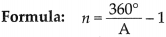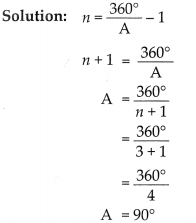The mirrors are inclined atan angle of 900 to each other.

Question 3.
An image is formed 5 cm behind a convex mirror of focal length 10 cm. At what distance is the object placed from the mirror?
Solution:
Given: Image distance (u) = 5 cm
Focal length (f) = 10 an
To find: Object distance (u) = ?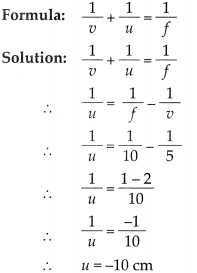The object is placed at a distance of 10 cm in front of the mirror.

Question 4.
An object placed 20 cm in front of a convex mirror is found to have an image 15cm behind the mirror. Find the focal length of the mirror.
Solution:
Given: Object distance (u) = -20 cm
Image distance (u) = 15 cm
To find: focal length (f) = ?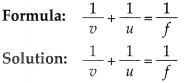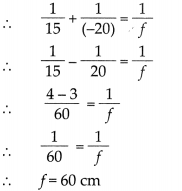The focal length of the convex mirror is 60 cm.

Numerical For Practice

Question 5.
An object is placed at a distance of 36 cm from a concave mirror of focal length 12 cm. Find the image distance.
-18 cm

Question 6.
An arrow is placed at a distance of 25 cm from a diverging mirror of focal length 20 cm. Find the image distance.
11.1 cm

Type – B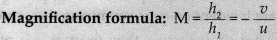Question 1.
An object 4cm in height is placed at a distance of 36 cm from a concave mirror. The image is formed 18 cm in the front of the mirror. Find the height of the image.
Solution:
Given: Object height (h1) = 4 cm
Image distance (u) = -18 cm
Object distance (u) = -36 cm
To find: Height of image (h2) = ?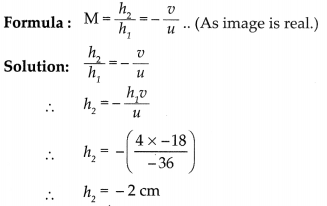The height of the image is 2 cm and it is inverted.

Question 2.
An object 2 cm high is placed at a distance of 16cm from a concave mirror which produces a real image 3 cm high. Find the image distance.
Solution:
Given: Object height (h1) = 2 cm
Object distance (u) = -16 cm
Image height (h2) = -3 cm
To find: Image distance (u) = ?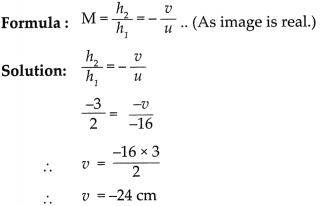The image is formed at a distance of 24 cm in front of the mirror.

Numericals For Practice

Question 3.
An object 10cm in height is placed at a distance of 36 cm from a concave mirror. 1f the image is formed at a distance of 18 cm in front of the mirror, find the height of the image.
-5cm

Question 4.
A converging mirror forms a real image of height 4 cm of an object of height 1 cm placed 20 cm away from the mirror. Find the image distance.
-80cm

Type – C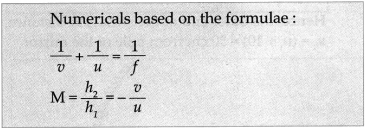Question 1.
Rajashree wants to get an inverted image of height 5 cm of an object kept at a distance of 30 cm from a concave mirror. The focal length of the mirror is 10 cm. At what distance from the mirror should she place the screen? What will be the type of the image, and what is the height of the object?
Solution:
Given:
Focal length = f = -10 cm,
Object distance = u = -30 cm
Height of the image = h2 = 7 cm
To find: Height of the object = h1 = ?
Image distance = u =?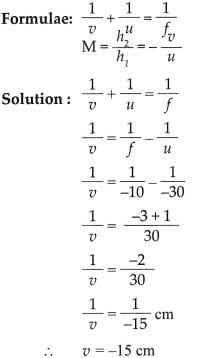Rajashree has to place the screen 15 cm to the left of the mirror.
Magnification formula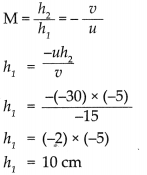The height of the object is 10 cm. Thus, the image will be real and diminished.

Question 2.
A 10 cm long stick is kept horizontally in front of the concave mirror having focal length of 10 cm in such a way that the end of the stick closest to the pole is at a distance of 20 cm. What will be the length of the image?
Solution:
The stick is kept parallel to the Principal axis. Distance between A and P is 20 cm. Say u1 = 20 cm.
Hence, the other end of the stick is at distance, u2 = (u1 + 10) = 30 cm from pole of the mirror.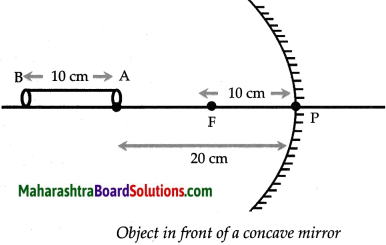Using mirror formula for concave mirror,
Solution: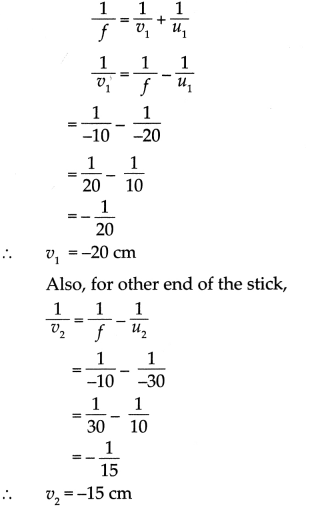Here, negative signs indicate that images are formed on the left of the mirror.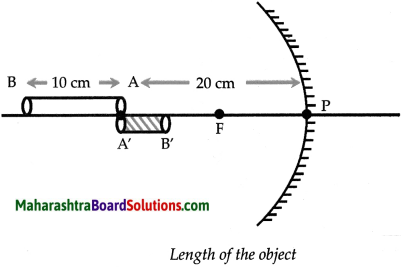The length of the image formed ?s given by, u = u2 – u1 = 15 – (-20) = 5cm.
The length of the image is 5 cm.

Numerical For Practice

Question 3.
An object 2 cm in height is placed at a distance of 16 cm from a concave mirror. If the focal length of the mirror is 9.6 cm., find the image distance, nature and size of the image.
u = -24 an, h2 = -3 cm; real, inverted and enlarged.

Question 4.
An arrow of 2.5cm height is placed at a distance of 25 cm from a diverging mirror of focal length 20 cm. Find the nature, position and size of the image formed.
v = 11.1cm, h= 1.1cm; virtual and in dimirrished form.

Define the following:

Question 1.
Centre of curvature of mirror (C)
Ans.
The centre of the sphere of which the mirror is a parte is called the centre of curvature of the mirror.

Question 2.
The radius of the sphere of which the mirror is a part, is called the radius of curvature of the mirror.

Question 3.
Pole (P)
The centre of the mirror surface is called its pole.

Question 4.
The principal axis of a mirror
The straight line passing through the pole and centre of curvature of the mirror is called its principal axis.

Question 5.
The focus of a concave mirror (F)
Incident rays which are parallel to the principal axis of a concave mirror, after reflection from the mirror, meet at a particular point in front of the mirror on the principal axis. This point (F) is called the principal focus of the concave mirror.

Question 6.
Focus of a convex mirror (F)
Incident rays parallel to the principal axis, after reflection, appear to come from a particular point behind the mirror lying along the principal axis. This point is called the principal focus of the convex mirror.

Question 7.
Focal length of a mirror (f)
The distance (f) between the pole and the principal focus of the mirror is called the focal length. This distance is half of the radius of curvature of the mirror. 𝑓=𝑅2

Question 1.
What are the rules for drawing ray diagrams for the formation of image by spherical mirror?
The rules are as follows :

• If an incident ray is parallel to the principal axis, then the reflected ray passes through the principal focus.
• If an incident ray passes through the principal focus of the mirror, the reflected ray is parallel to the principal axis.
• If an incident ray passes through the centre of curvature of the mirror, the reflected ray traces the same path back.

Distinguish between:

Question 1.
Convex mirror and Concave mirror

 Convex mirror Concave mirror (i) In a convex mirror, the reflecting surface is on the outer side.(ii) It is called a diverging mirror.(iii) The focus of a convex mirror is virtual.(iv) It can form only a virtual image.(v) It can form only a diminished image. (i) In a concave mirror, the reflecting surface is on the inner side.(ii) It is called a converging mirror.(iii) The focus of a concave mirror is real.(iv) It can form a real as well as a virtual image.(v) It can form an enlarged, diminished as well as the same size image.

Question 2.
Real image and Virtual image

 Real image Virtual image (i) A real image is formed only when the reflected rays actually meet at a point.(ii) Real images can be obtained on a screen.(iii) All real images are inverted. (i) A virtual image is formed only when the reflected rays appear to meet at a point.(ii) Virtual images cannot be obtained on a screen.(iii) All virtual images are erect.

Question 1.
If we keep the mirrors parallel to each other, how many images will we see ?
When two mirrors are kept parallel to each other infinite images are formed, this is because light gets reflected infinite times.

Question 1.
What sign conventions are used for reflection from a spherical mirror?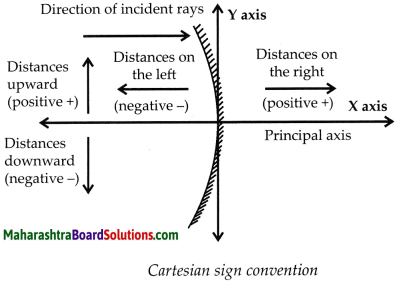According to the Cartesian sign convention, the pole of the mirror is taken as the origin. The principal axis is taken as the X-axis of the frame of reference. The sign conventions are as follows.

• The object is always kept on the left of the mirror. All distances parallel to the principal axis are measured from the pole of the mirror.
• All distances measured towards the right of the pole are taken to be positive, while those measured towards the left are taken to be negative.
• Distance measured vertically upwards from the principal axis are taken to be positive.
• Distance measured vertically downwards from the principal axis are taken to be negative.
• The focal length of a concave mirror is negative while that of a convex mirror is positive.

Question 2.
Draw ray diagrams for the image obtained in convex mirrors.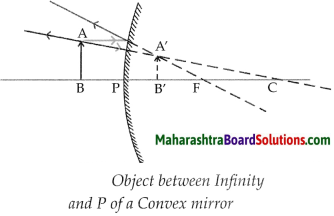Image position Nature of image Behind the mirror. (A) Virtual,(B) Erect(C) Diminished

Question 3.
In order to see the full image of a person standing in front of a mirror, the minimum height of the mirror must be half the height of the person. Explain.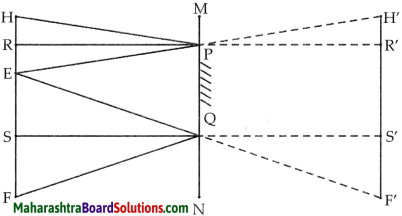Proof:

1. In the figure, the point at the top of the head, the eyes and a point at the feet of a person are indicated by H, E and F respectively.
2. R and S are midpoints of HE and EF respectively.
3. The mirror PQ is at a height of NQ from the ground and is perpendicular to it. PQ is the minimum height of the mirror in order to obtain the full image of the person.

For this, RP and QS must be perpendicular to the mirror.

Minimum height of the mirror
PQ = RS
= RE + ES
=HE2+EF2=HF2
= Half of the person’s height.

Question 4.
Determine the sign of magnification in each of the 6 cases in the table and verify that they are same using formulae
𝐌=ℎ2ℎ1 and 𝐌=−𝑣𝑢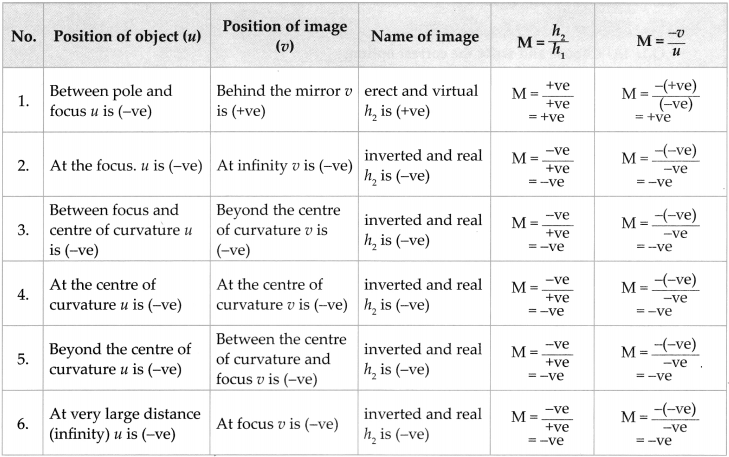Question 5.
Explain the images formed by concave mirrors with respect to position of the image and object and also the Nature and size of image## निष्कर्ष

आम्हाला आशा आहे की महाराष्ट्रावरील हा लेख तुम्हाला 'नववी विज्ञान धडा प्रकाशाचे प्रतिबिंब स्वाध्याय' मदत करेल. तुम्हाला काही प्रश्न असल्यास, त्यांना खाली टिप्पणी विभागात मोकळ्या मनाने पोस्ट करा. आम्ही लवकरात लवकर तुमच्याकडे परत येऊ.
Share: